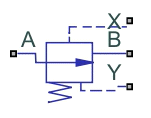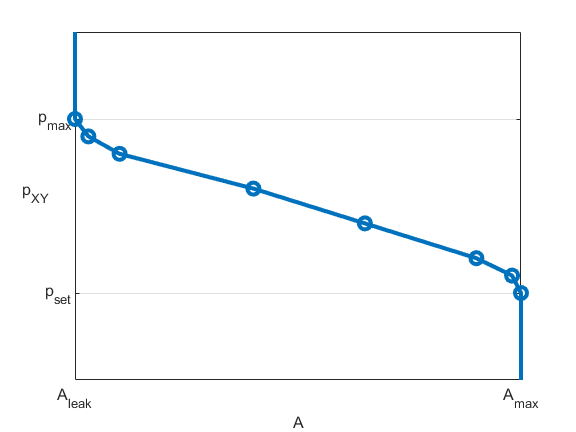# Pressure Compensator Valve (IL)

Pressure compensator valve in an isothermal liquid network

Since R2020a

•Libraries:
Simscape / Fluids / Isothermal Liquid / Valves & Orifices / Pressure Control Valves

## Description

The Pressure Compensator Valve (IL) block represents a pressure compensator in an isothermal liquid network, such as a pressure relief valve or pressure-reducing valve. Use this valve to maintain the pressure at the valve based on signals from another part of the system.

The pressure differential between ports X and Y is the control pressure, Pcontrol. When this value meets or exceeds the set pressure, the valve area opens or closes depending on the Valve specification parameter. The pressure regulation range begins at the set pressure, Pset. You can choose between constant and controlled set pressure regulation. A physical signal at port Ps provides a varying set pressure.

### Pressure Control

The block regulates pressure when Pcontrol exceeds Pset. The block continues to regulate the pressure up to Pmax, the sum of Pset and the pressure regulation range. The block supports two modes of regulation:

• When you set Set pressure control to `Controlled` and connect a pressure signal to port Ps, the block keeps the pressure regulation range constant. The valve regulates pressure when Pcontrol is greater than the value of the signal at port Ps and less than Pmax.

• When you set Set pressure control to `Constant`, the Set pressure differential parameter defines a constant set pressure.

### Conservation of Mass

The block conserves mass such that

`${\stackrel{˙}{m}}_{A}+{\stackrel{˙}{m}}_{B}=0.$`

The block calculates the mass flow rate through the valve as

`$\stackrel{˙}{m}=\frac{{C}_{d}{A}_{valve}\sqrt{2\overline{\rho }}}{\sqrt{P{R}_{loss}\left(1-{\left(\frac{{A}_{valve}}{{A}_{port}}\right)}^{2}\right)}}\frac{\Delta p}{{\left[\Delta {p}^{2}+\Delta {p}_{crit}^{2}\right]}^{1/4}},$`

where:

• Cd is the Discharge coefficient parameter.

• Avalve is the instantaneous valve open area.

• Aport is the Cross-sectional area at ports A and B parameter.

• $\overline{\rho }$ is the average fluid density.

• Δp is the valve pressure difference pApB.

The critical pressure difference, Δpcrit, is the pressure differential specified by the Critical Reynolds number parameter, Recrit. This parameter represents the flow regime transition point between laminar and turbulent flow. The block finds the critical pressure difference as

`$\Delta {p}_{crit}=\frac{\pi \overline{\rho }}{8{A}_{valve}}{\left(\frac{\nu {\mathrm{Re}}_{crit}}{{C}_{d}}\right)}^{2}.$`

The pressure loss, PRloss, describes the reduction of pressure in the valve due to a decrease in area. The block calculates the pressure loss as:

`$P{R}_{loss}=\frac{\sqrt{1-{\left(\frac{{A}_{valve}}{{A}_{port}}\right)}^{2}\left(1-{C}_{d}^{2}\right)}-{C}_{d}\frac{{A}_{valve}}{{A}_{port}}}{\sqrt{1-{\left(\frac{{A}_{valve}}{{A}_{port}}\right)}^{2}\left(1-{C}_{d}^{2}\right)}+{C}_{d}\frac{{A}_{valve}}{{A}_{port}}}.$`

The pressure recovery describes the positive pressure change in the valve due to an increase in area. When you set Pressure recovery to `Off`, the block sets PRloss to 1.

The block calculates Avalve using the opening parameterization and the valve opening dynamics.

### Valve Opening Parameterization

When you set Opening parameterization to `Linear`, the valve area for normally open valves is

`${A}_{valve}=\stackrel{^}{p}\left({A}_{leak}-{A}_{max}\right)+{A}_{max}.$`

This figure demonstrates how the block controls the opening area for a normally open valve using the linear parameterization.For normally closed valves, the block uses

`${A}_{valve}=\stackrel{^}{p}\left({A}_{max}-{A}_{leak}\right)+{A}_{leak}.$`

This figure demonstrates how the block controls the opening area for a normally closed valve using the linear parameterization.The normalized pressure, $\stackrel{^}{p}$, is

`$\stackrel{^}{p}=\frac{{p}_{control}-{p}_{set}}{{p}_{max}-{p}_{set}}.$`

When the valve is in a near-open or near-closed position in the linear parameterization, you can maintain numerical robustness in your simulation by adjusting the parameter. If the parameter is nonzero, the block smoothly saturates the control pressure between pset and pmax. For more information, see Numerical Smoothing.

When you set Opening parameterization to ```Tabulated data```, Aleak and Amax are the first and last parameters of the Opening area vector parameter, respectively. The block calculates the opening area as

`${A}_{valve}=tablelookup\left({p}_{control,TLU,ref},{A}_{TLU},{p}_{control},interpolation=linear,extrapolation=nearest\right),$`

where:

• pcontrol is the control pressure, the pressure differential between ports X and Y.

• pcontrol,TLU,ref = pTLU + poffset.

• pTLU is the Pressure differential vector parameter.

• poffset is an internal pressure offset that causes the valve to start closing when pcontrol,TLU,ref = pset.

• ATLU is the Opening area vector parameter.

This figure demonstrates how the block controls the opening area for a normally open valve using the tabulated parameterization.This figure demonstrates how the block controls the opening area for a normally closed valve using the tabulated parameterization.### Opening Dynamics

When you select Opening dynamics, the block introduces a control pressure lag where pcontrol becomes the dynamic control pressure, pdyn. The block calculates the instantaneous change in dynamic control pressure based on the Opening time constant parameter, τ:

`${\stackrel{˙}{p}}_{dyn}=\frac{{p}_{control}-{p}_{dyn}}{\tau }.$`

By default, the block does not model Opening dynamics. For the linear parameterization, a nonzero value for the Smoothing factor parameter provides additional numerical stability when the orifice is in near-closed or near-open position.

The block calculates the steady-state dynamics according to the Opening parameterization parameter, and are based on the control pressure, pcontrol.

### Faults

When you set Enable faults to `On`, the valve open area stops at a specified value in response to one of these triggers:

• Simulation time — The fault occurs at a specified time during the simulation.

• Simulation behavior — The fault occurs in response to an external trigger.

You can set the Opening area when faulted parameter to:

• `Closed` — The valve area stops at its smallest value, depending on the Opening parameterization parameter setting:

• `Linear` — The valve area stops at the value of the Leakage area parameter.

• `Tabulated data` — The valve area stops at the smallest element of the Opening area vector parameter.

• `Open` — The valve stops at its largest value, depending on the Opening parameterization parameter setting:

• `Linear` — The valve area stops at the value of the Maximum opening area parameter.

• `Tabulated data` — The valve area stops at the largest element of the Opening area vector parameter.

• `Maintain last value` — The valve area stops at the valve open area when the trigger occurred.

Due to numerical smoothing at the extremes of the valve area, the minimum area applied is larger than the leakage area, and the maximum is smaller than the value of the Maximum orifice area parameter. This effect is in proportion to the amount of smoothing you apply.

After the fault triggers, the valve remains at the faulted area for the rest of the simulation.

## Ports

### Conserving

expand all

Isothermal liquid conserving port associated with the A side of the valve.

Isothermal liquid conserving port associated with the B side of the valve.

Isothermal liquid conserving port associated with sensing the pressure at point X, Px.

Isothermal liquid conserving port associated with point Y, Py.

### Input

expand all

Pressure differential for controlled valve operation, in Pa.

#### Dependencies

To enable this port, set Set pressure control to `Controlled`.

Physical signal port for an external fault trigger, unitless. Triggering occurs when the value is greater than 0.5.

#### Dependencies

To enable this port, set Enable faults to `On` and Fault trigger to `External`.

## Parameters

expand all

### Parameters

Normal operating condition of the pressure compensator valve. For a reducing valve, choose `Normally open` valve. For a relief valve, choose `Normally closed`.

Valve operation method. When you set this parameter to:

• `Controlled` and connect a pressure signal to port Ps, the block keeps the pressure regulation range constant. The valve regulates pressure when Pcontrol is greater than the value of the signal at port Ps and less than Pmax.

• `Constant`, the Set pressure differential parameter defines a constant set pressure.

Method to parameterize the opening action of the valve.

Magnitude of the pressure differential that triggers pressure compensation.

#### Dependencies

To enable this parameter, set Set pressure control to `Constant`.

Operational pressure range of the valve. The pressure regulation range defines the difference between the Set pressure differential parameter and the maximum valve operating pressure.

#### Dependencies

To enable this parameter, set Opening parameterization to `Linear`.

Cross-sectional area of the valve in the fully open position.

#### Dependencies

To enable this parameter, set Opening parameterization to `Linear`.

Sum of all gaps when the valve is in the fully closed position. Any area smaller than this value saturates to the specified leakage area. This value contributes to numerical stability by maintaining continuity in the flow.

#### Dependencies

To enable this parameter, set Opening parameterization to `Linear`.

Vector of pressure differential values for the tabulated parameterization of the opening area. The vector elements must correspond one-to-one to the values in the Opening area vector parameter. The pressures must be in ascending order.

#### Dependencies

To enable this parameter, set Opening parameterization to ```Tabulated data```.

Vector of opening area values for the tabulated parameterization of the opening area. The vector elements must correspond one-to-one to the values in the Pressure differential vector parameter. For normally open valves, list elements in descending order. For normally closed valves, list elements in ascending order.

The opening area vector must have the same number of elements as the Pressure differential vector. The block uses linear interpolation between table data points.

#### Dependencies

To enable this parameter, set Opening parameterization to ```Tabulated data```.

Cross-sectional area at the entry and exit ports A and B. The block uses this area in the pressure-flow rate equation that determines mass flow rate through the valve.

Correction factor that accounts for discharge losses in theoretical flows.

Upper Reynolds number limit for laminar flow through the valve.

Whether to account for pressure increase when fluid flows from a region of a smaller cross-sectional area to a region of larger cross-sectional area.

Continuous smoothing factor that introduces a layer of gradual change to the flow response when the valve is in near-open or near-closed positions. Set this value to a nonzero value less than one to increase the stability of your simulation in these regimes.

#### Dependencies

To enable this parameter, set Opening parameterization to `Linear`.

Whether to account for transient effects to the fluid system due to the valve opening. When you select Opening dynamics, the block approximates the opening conditions by introducing a first-order lag in the flow response.

Time constant by which to compute the lag in the opening dynamics.

#### Dependencies

To enable this parameter, select Opening dynamics.

### Faults

Whether to enable externally or temporally triggered faults. After a fault, the block sets the valve area to the value specified in the Opening area when faulted parameter.

Opening area that the valve assume following a fault. You can choose for the valve to seize when the valve is opened, closed, or at the area after a fault.

#### Dependencies

To enable this parameter, set Enable faults to `On`.

Whether a fault trigger occurs due to an external event or at a specified time. When you select:

• `External` — The block enables port Tr. A physical signal at port Tr that is greater than `0.5` triggers faulting.

• `Temporal` — When the simulation time reaches the value of the Simulation time for fault event parameter, the block sets the valve area according to the Opening area when faulted parameter.

#### Dependencies

To enable this parameter, set Enable faults to `On`.

When the simulation time reaches the value of the Simulation time for fault event parameter, the block sets the valve area according to the Opening area when faulted parameter.

#### Dependencies

To enable this parameter, set Enable faults to `On` and Fault trigger to `Temporal`.

## Version History

Introduced in R2020a